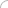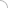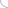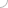# DBMS interview questions and answers

## DBMS interview questions and answers

### DBMS interview test (20 questions) RDBMS interview questions and answers QUESTION - Primitive operations common to all record management system include ? -Print -Sort -Look up -All of above View Answer / Hide Answer ANSWER - Look up QUESTION - The master list of an indexed file ? -Is sorted in ascending order -contains only a list of keys and record numbers -has a number assigned to each record -Both B and C View Answer / Hide Answer ANSWER - has a number assigned to each record QUESTION - A condition that led to the development of database was ? -A demand for more data to support information needs -An increase in the amount of data handled by organizations -The poliferation of data files -All of above View Answer / Hide Answer ANSWER - All of above QUESTION - A relation scheme is said to be in ______________ form if the values in the domain of each attribute of the relation are atomic ? -Unnormalized -First Normal -BoyceCODD -None of these View Answer / Hide Answer ANSWER - First Normal QUESTION - A second Normal form does not permit ______________ dependency between a non prime attribute and the relation key ? -Partial -Multi -Functional -Valued View Answer / Hide Answer ANSWER - Partial QUESTION - A relation scheme is in ____________ if it is in the 1NF and if all non key attributes are fully functionally dependent on the primary key ? -First Normal Form -Second Normal Form -Boyce Codd Normal Form -Fourth Normal Form View Answer / Hide Answer ANSWER - Second Normal Form QUESTION - Administrate supervision of database activity is the responsibility of ? -Database administrator -DP Manager -DB Manager -DP Administration View Answer / Hide Answer ANSWER - Database administrator QUESTION - Data security threats include ? -hardware failure -privacy invasion -fraudulent manipulation of data -All of above View Answer / Hide Answer ANSWER - privacy invasion QUESTION - Mechanism developed to enforce users to enter data in required format is: -Data validation -Input mask -Criteria -Data verification View Answer / Hide Answer ANSWER - Input mask QUESTION - Association between entities is represented by ____ shape -Rectangle -Diamond -Double Rectangle -Ellipse View Answer / Hide Answer ANSWER - Diamond QUESTION - _______ depicted as ellipses and labeled with the name of the property -Aggregation -Attributes -Entities -Relationships View Answer / Hide Answer ANSWER - Attributes QUESTION - Entity relationship model is introduced by -Peter Chen -Larry Ellison -Charles Bachman -E.F. Codd View Answer / Hide Answer ANSWER - Peter Chen QUESTION - Which of the following helps in achieving a good database design? -Each table should have an identifier -Columns that contain NULL values should be created -A table should store data for all the related entities together -Columns that contain NULL values should not be created View Answer / Hide Answer ANSWER - Each table should have an identifier QUESTION - For which normal form you need to remove non key attributes that are functionally dependent on attributes that are not primary key? -First Normal Form -Third Normal Form -Second Normal Form -Boyce Codd First Normal Form View Answer / Hide Answer ANSWER - Third Normal Form QUESTION - The attribute B is fully functionally dependent on the attribute A if -Each value of A determines one and only one value of B -Each value of A determines at least one value of B -Each value of A determines all values of B -Each value of A determines either one or more values of B View Answer / Hide Answer ANSWER - Each value of A determines one and only one value of B QUESTION - An __________ lock is issued when a transaction wants to update(write) a data item and no locks are currently held on that data item by any other transaction -Exclusive -Shared -No Lock -Binary View Answer / Hide Answer ANSWER - Exclusive QUESTION - A levels of abstraction which describes data stored in database, and the relationships among the data is -Physical level -Logical level -View level -None of the above View Answer / Hide Answer ANSWER - Logical level QUESTION - For which normal form you need to remove non key attributes that are functionally dependent on attributes that are not primary key? -First Normal Form -Third Normal Form -Second Normal Form -Boyce Codd First Normal Form View Answer / Hide Answer ANSWER - Third Normal Form QUESTION - Schema is viewed as a Graph data structure in _________data model -Network -Hierarchical -Object Oriented -Relational View Answer / Hide Answer ANSWER - Network QUESTION - The attribute B is fully functionally dependent on the attribute A if -Each value of A determines one and only one value of B -Each value of A determines at least one value of B -Each value of A determines all values of B -Each value of A determines either one or more values of B View Answer / Hide Answer ANSWER - Each value of A determines one and only one value of B QUESTION - If there is a functional dependency between two columns, A and B , such that B is functionally dependent on A and there is functional dependency between two columns B and c such that C is functionally dependent on B then we can say that C is _____________ depend on A -Partially -Fully -Transitively -Not View Answer / Hide Answer ANSWER - Transitively QUESTION - Which is correct about physical data independence -ability to modify the physical schema without changing the logical schema -ability to modify the physical schema with changing the logical schema -ability to modify the physical schema without changing the view level schema -ability to modify the logical schema without changing the physical schema View Answer / Hide Answer ANSWER - ability to modify the physical schema without changing the logical schema QUESTION - The ability to change the logical schema without changing the external schema or application programs is called as Logical Data Independence -True -False View Answer / Hide Answer ANSWER - True (adsbygoogle = window.adsbygoogle || []).push({}); Write your comment - Share Knowledge and Experience (adsbygoogle = window.adsbygoogle || []).push({}); (adsbygoogle = window.adsbygoogle || []).push({});Interview questionsLatest MCQs » General awareness - Banking » ASP.NET » PL/SQL » Mechanical Engineering » IAS Prelims GS » Java » Programming Language » Electrical Engineering » English » C++ » Software Engineering » Electronic Engineering » Quantitative Aptitude » Oracle » English » Finance Home | About us | Sitemap | Contact us | We are hiring © Copyright 2016. All Rights Reserved. Terms of use  |  Follow us on Facebook!